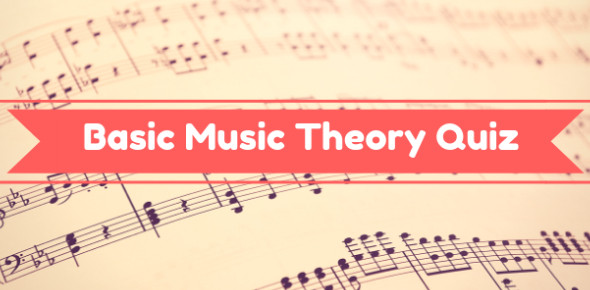# Music Theory Quiz 1

5 Questions | Attempts: 2456SettingsMusic being a very practical subject, it has the theory part which forms the basis of understanding and knowledge of music. Music theory quiz 1 tests on among other concepts these fundamental music basics. All the best.

• 1.
How many beats does a quarter note take?
• A.

Three

• B.

One

• C.

Half

• D.

Two

• E.

Four

• 2.
How many beats does a half note take?
• A.

Three

• B.

One

• C.

Half

• D.

Two

• E.

Four

• 3.
How many beats does a whole note take?
• A.

Three

• B.

One

• C.

Half

• D.

Two

• E.

Four

• 4.
How many beats does an eighth note take?
• A.

Three

• B.

One

• C.

Half

• D.

Two

• E.

Four

• 5.
When you look at a time signature, the top number shows how many _____ are in a measure.

## Related TopicsBack to top
×

Wait!
Here's an interesting quiz for you.